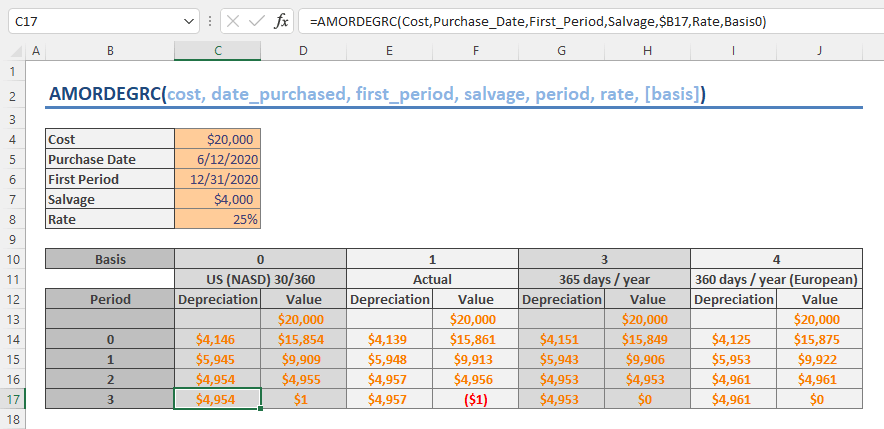The AMORDEGRC function is a Finance function used in French accounting system that returns the depreciation for each accounting period, on a prorated basis. The prorated depreciation is considered when an asset is purchased in the middle of the accounting period. In this guide, we’re going to show you how to use the AMORDEGRC function and go over some tips and error handling methods.

• All versions

## AMORDEGRC Function Syntax

AMORDEGRC(cost, date_purchased, first_period, salvage, period, rate, [basis])

## Arguments

 cost The initial cost of the asset. date_purchased The purchase date of the asset. first_period The end date of the first period. salvage The value after the asset has been fully depreciated, salvage value. period Specific period to calculation depreciation for. rate The rate of depreciation. [basis] Optional. The year basis type. Detailed information on Wikipedia.

### Basis

 Basis Date system 0 or omitted 360 days (NASD method) 1 Actual 3 365 days in a year 4 360 days in a year (European method)

## AMORDEGRC Function Examples

In our example, an asset was purchased on June 12, 2020 at an initial cost of \$20,000. The end of the first period is December 31, 2020 and the depreciation rate is 20% per year with an expected salvage value of \$4,000.

The table below shows the depreciation and asset value over periods for each basis.## Remarks

• Microsoft suggests using the DATE function or other date returning functions for date_purchased and first_period arguments instead of strings.
• The AMORDEGRC applies a depreciation coefficient in the calculation based on the life of the asset.
 Asset life Depreciation coefficient Between 3 and 4 years 1.5 Between 5 and 6 years 2 More than 6 years 2.5
• Use the AMORLINC formula to calculate depreciation without applying a coefficient.

## Excel Depreciation Functions

 Straight line SLN Sum-of-years' digits SYD Fixed-declining balance DB Double-declining balance DDB Variable-declining balance VDB French straight line AMORLINC